# Electronics and Communication Engineering - Analog Electronics

1.
To prevent a DC return between source and load, it is necessary to use
either (a) or (b)
Explanation:

Capacitor offers infinite impedance to DC.

2.
For a base current of 10 μA, what is the value of collector current in common emitter if βdc = 100
10 μA
100 μA
1 mA
10 mA
Explanation:

IC = 10 x 100 μA = 1 mA.

3.
Which of the following oscillators is suitable for frequencies in the range of mega hertz?
RC phase shift
Wien bridge
Hartley
Both (a) and (c)
Explanation:

Only LC oscillators are suitable for MHz range.

4.
If the input to the ideal comparator shown in the figure is a sinusoidal signal of 8 V (peak to peak) without any DC component, then the output of the comparator has a duty cycle of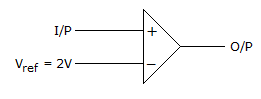1/2
1/3
1/6
1/12
Explanation: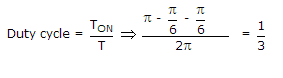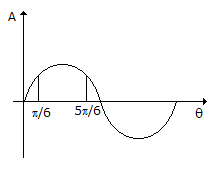5.
A half wave diode circuit using ideal diode has an input voltage 20 sin ωt volts. Then average and rms values of output voltage are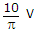and 10 V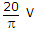and 10 Vand 5 Vand 5 V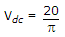and VC = 0.5 x 20 = 10 V.